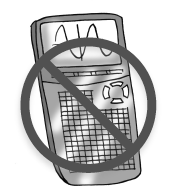### Home > INT1 > Chapter 10 > Lesson 10.1.3 > Problem10-46

10-46.

An auto repair shop is concerned about the reliability of its brake lathe. A lathe is a rotating piece of equipment used in restoring parts. The shop’s owner measured the rotations per minute (rpm) at various times over the course of a month. These were his measurements: Homework Help ✎$250$, $251$, $253$, $253$, $253$, $254$, $257$, $257$, $259$, $259$, $261$, $263$, $265$, $270$, $291$ rpm

1. On grid paper, draw a combination histogram and boxplot. Do not use a calculator.

Consider what an appropriate interval size would be for this data set.

1. Describe center, shape, spread, and outliers, similar to the way you did in problem 10‑44.

Review the work you did on problem 10-44.

2. When reporting the center of this data, or the “typical rpm,” which would be more appropriate, the mean or the median? Why?

The median ($257$) is probably the more appropriate descriptor of the center.
This is because the outlier of $291$ has skewed the data some.
Removing the outlier causes the mean and the median to be nearly the same.